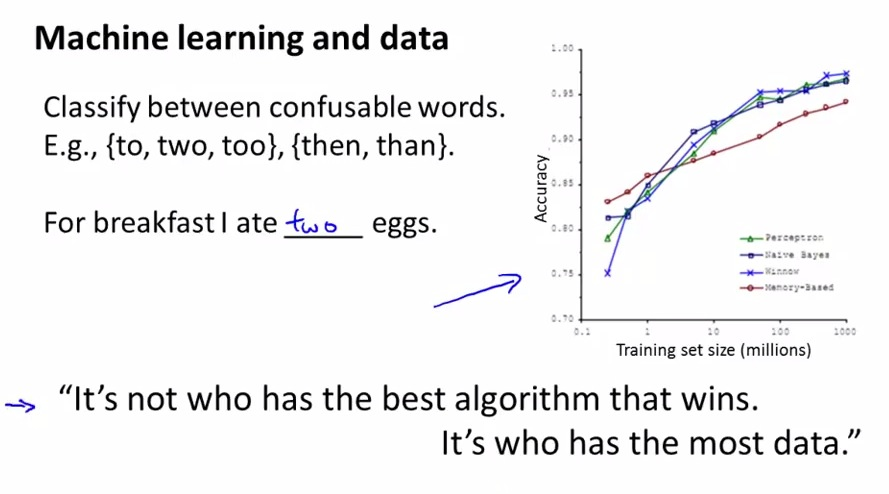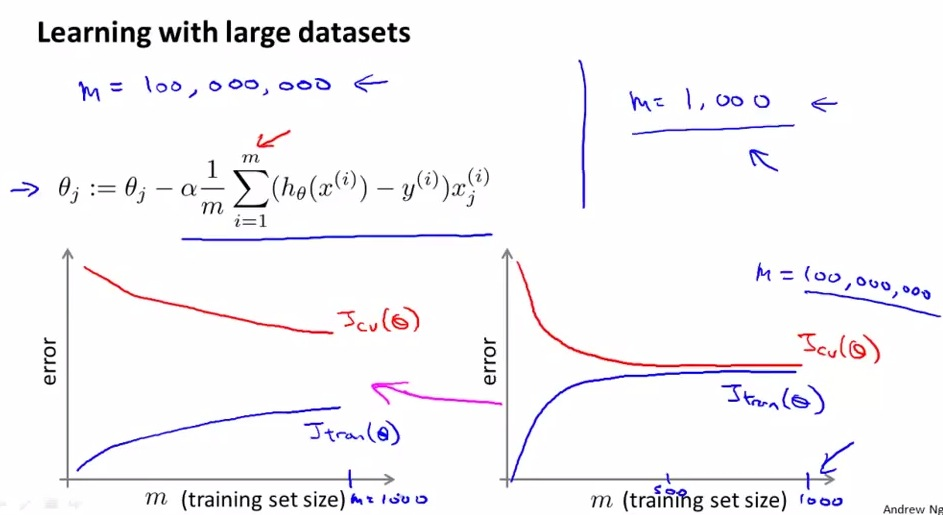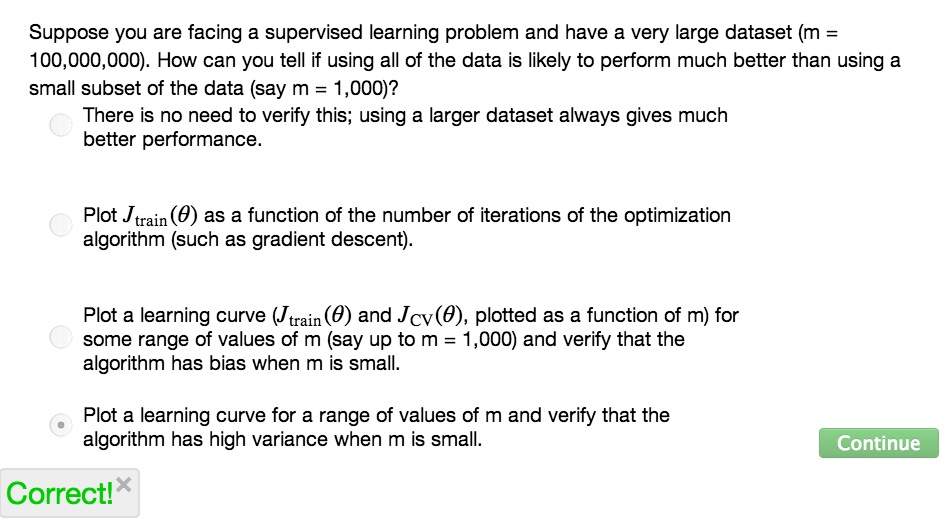• This section of video we are trying to come up with a solution when facing much larger datasets
• If we review our past ten years back with machine learning, only the datasets that keep increasing
• This section we try to make deduction when handling massive data• This is example of confusable words earlier
• Recall of what the scientist are saying• Sometimes, we'll end up facing with hundreds of millions of datasets. This is surely become highly computational expensive. It becomes more expensive, if we try to recursive. At the end of this lesson we will know how to replace this recursive method with something that more efficient
• But before that, let's think about the datasets. Is it really benefit from increasing much larger data? Why not first take the 1K examples, the good sanity check
• Then we may want to put our usual leaning curve, put the 1K examples and see the curve
• If the graph will be like on the left, then it's the high variance example.So maybe increasing additional data will be likely to help
• On the contrary, the figure on the right is the high bias example. Then adding additional data is not the solution. We may want to add more features and see if the learning algorithm works really well with the learning curve.
• Adding extra features, slightly move to the figure like in the left, take a deeper look also if the data has high variance.• In summary, we know what's the first step when handling much larger datasets, try to make sanity check whether or not increasing the data will be likely to help
• In the next video, we learn about Stochastic Gradient and MapReduce, the solution when handling much larger data sets, to scale our learning algorithm with big data